# Example 2: Comparing two standard error estimators

This vignette is adapted from Example 2 on the SimEngine website.

library(tidyr)
library(SimEngine)

When developing a novel statistical method, we often wish to compare our proposed method with one or more existing methods. This serves to highlight the differences between our method and whatever is used in common practice. Generally, we wish to examine realistic settings, motivated by statistical theory, in which the novel method confers some advantage over the alternatives.

In this example, we will consider the problem of estimating the variance-covariance matrix of the least-squares estimator in linear regression. We assume the reader has some familiarity with linear regression.

Suppose our dataset consists of $$n$$ independent observations $$\{(Y_1, X_1), \dots, (Y_n, X_n)\}$$, where $$X$$ and $$Y$$ are both scalar variables. A general linear regression model posits that

$Y_i = \beta_0 + \beta_1 X_i + \epsilon_i$

where $$\epsilon_i$$ is a mean-zero noise term with variance $$\sigma^2_i$$. We refer to this as a heteroskedastic model, since the variances need not be equal across all $$i$$. This is the true data-generating model. (Note: A more restrictive (but misspecified) model assumes that there is a common variance $$\sigma^2$$ such that $$\sigma^2_i = \sigma^2$$ for all $$i$$. We refer to this incorrect model as the homoskedastic model.)

In linear regression, we use least-squares to estimate the coefficient vector $$\beta$$. For the purposes of building confidence intervals and performing hypothesis tests, we need to also estimate the standard error of the least squares estimator. There are two common ways to do this: (1) Use a model-based standard error that assume homoskedasticity. This is the estimator used by default in most statistical software (including the lm() function in R). (2) Use a so-called sandwich standard error. Statistical theory tells us that estimator will be consistent even under heteroskedasticity. See the Statistical Appendix for more details.

We will carry out a small simulation study to compare these two estimators.

We start by declaring a new simulation object and writing a function that generates some data according to our model. For this simulation, we will make $$\sigma^2_i$$ larger for larger values of $$X_i$$.

sim <- new_sim()

create_regression_data <- function(n) {
beta <- c(-1, 10)
x <- rnorm(n)
sigma2 <- exp(x)
y <- rnorm(n=n, mean=(beta+beta*x), sd = sqrt(sigma2))
return(data.frame(x=x, y=y))
}

To get a sense of what heteroskedasticity looks like in practice, we can generate a dataset, fit a linear regression model, and make a scatterplot of the residuals against $$X$$.

dat <- create_regression_data(n=500)
linear_model <- lm(y~x, data=dat)
dat$residuals <- linear_model$residuals

library(ggplot2)
ggplot(dat, aes(x=x, y=residuals)) +
geom_point() +
theme_bw() +
labs(x="x", y="residual")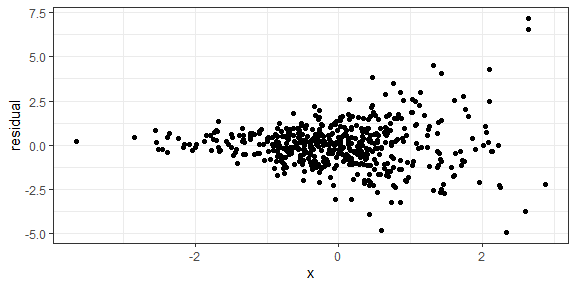Now we declare two methods: one returns the least squares estimate and model-based estimator of the variance-covariance matrix of $$\hat{\beta}$$, and the other returns the least squares estimate and sandwich estimator (using the sandwich package).

model_vcov <- function(data) {
mod <- lm(y~x, data=data)
return(list("coef"=mod$coefficients, "vcov"=diag(vcov(mod)))) } sandwich_vcov <- function(data) { mod <- lm(y~x, data=data) return(list("coef"=mod$coefficients, "vcov"=diag(vcovHC(mod))))
}

Next, we write the simulation script. This script returns a point estimate and a standard error estimate for both the intercept parameter $$\beta_0$$ and the slope parameter $$\beta_1$$. We will tell SimEngine to run 500 simulation replicates for each of four sample sizes. It is important to use the seed argument in set_config so that our results will be reproducible. In addition, we will use the packages option to load the sandwich package. Loading packages via set_config (as opposed to running library(sandwich)) is required if running simulations in parallel. Finally, we run the simulation.

sim %<>% set_script(function() {
data <- create_regression_data(n=L$n) estimates <- use_method(L$estimator, list(data))
return(list(
"beta0_est" = estimates$coef, "beta1_est" = estimates$coef,
"beta0_se_est" = sqrt(estimates$vcov), "beta1_se_est" = sqrt(estimates$vcov)
))
})

sim %<>% set_levels(
estimator = c("model_vcov", "sandwich_vcov"),
n = c(50, 100, 500, 1000)
)

sim %<>% set_config(
num_sim = 500,
seed = 24,
packages = c("sandwich")
)

sim %<>% run()
#> Done. No errors or warnings detected.

Now we can summarize the results using summarize. There are two main quantities of interest for us. The primary purpose of the standard error estimate for $$\hat{\beta}$$ is to form confidence intervals, so we will look at (1) the average width of the resulting interval (simply 1.96 times the average standard error estimate across simulation replicates), and (2) the estimated coverage of the interval, which is simply the proportion of simulation replicates in which the interval contains the true value of $$\beta$$. We will focus on 95% confidence intervals in this simulation.

summarized_results <- sim %>% summarize(
mean = list(
list(name="mean_se_beta0", x="beta0_se_est"),
list(name="mean_se_beta1", x="beta1_se_est")
),
coverage = list(
list(name="cov_beta0", estimate="beta0_est", se="beta0_se_est", truth=-1),
list(name="cov_beta1", estimate="beta1_est", se="beta1_se_est", truth=10)
)
)

print(summarized_results)
#>   level_id     estimator    n mean_se_beta0 mean_se_beta1 cov_beta0 cov_beta1
#> 1        1    model_vcov   50    0.18110782    0.18232629     0.948     0.850
#> 2        2 sandwich_vcov   50    0.18229941    0.24313215     0.938     0.922
#> 3        3    model_vcov  100    0.12720997    0.12764561     0.946     0.864
#> 4        4 sandwich_vcov  100    0.12800377    0.17264265     0.942     0.946
#> 5        5    model_vcov  500    0.05703074    0.05697449     0.946     0.836
#> 6        6 sandwich_vcov  500    0.05749570    0.07968853     0.948     0.942
#> 7        7    model_vcov 1000    0.04055713    0.04058957     0.962     0.858
#> 8        8 sandwich_vcov 1000    0.04052118    0.05721305     0.958     0.958

To visualize our results, we set up a plotting function.

plot_results <- function(which_graph, n_est) {
if (n_est == 3) {
values <- c("#999999", "#E69F00", "#56B4E9")
breaks <- c("model_vcov", "sandwich_vcov", "bootstrap_vcov")
labels <- c("Model-based", "Sandwich", "Bootstrap")
} else {
values <- c("#999999", "#E69F00")
breaks <- c("model_vcov", "sandwich_vcov")
labels <- c("Model-based", "Sandwich")
}
if (which_graph == "width") {
summarized_results %>%
pivot_longer(
cols = c("mean_se_beta0", "mean_se_beta1"),
names_to = "parameter",
names_prefix = "mean_se_"
) %>%
dplyr::mutate(value_j = jitter(value, amount = 0.01)) %>%
ggplot(aes(x=n, y=1.96*value_j, color=estimator)) +
geom_line(aes(linetype=parameter)) +
geom_point() +
theme_bw() +
ylab("Average CI width") +
scale_color_manual(
values = values,
breaks = breaks,
name = "SE estimator",
labels = labels
) +
scale_linetype_discrete(
breaks = c("beta0", "beta1"),
name = "Parameter",
labels = c(expression(beta), expression(beta))
)
} else {
summarized_results %>%
pivot_longer(
cols = c("cov_beta0","cov_beta1"),
names_to = "parameter",
names_prefix = "cov_"
) %>%
dplyr::mutate(value_j = jitter(value, amount = 0.01)) %>%
ggplot(aes(x=n, y=value, color=estimator)) +
geom_line(aes(linetype = parameter)) +
geom_point() +
theme_bw() +
ylab("Coverage") +
scale_color_manual(
values = values,
breaks = breaks,
name = "SE estimator",
labels = labels
) +
scale_linetype_discrete(
breaks = c("beta0", "beta1"),
name = "Parameter",
labels = c(expression(beta), expression(beta))
) +
geom_hline(yintercept=0.95)
}
}

We then use the plotting function to make our figures.

plot_results("width", 2)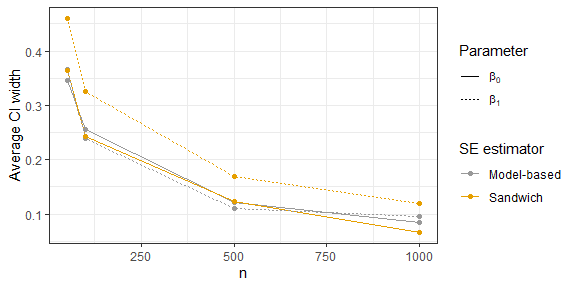plot_results("coverage", 2)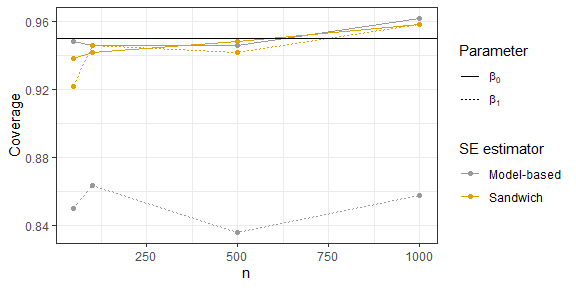Looking at these plots, we can see that the sandwich method results in a wider interval, on average, for $$\beta_1$$. In terms of coverage, the sandwich estimator achieves near nominal coverage for both parameters, while there is moderate undercoverage for $$\beta_1$$ using the model-based estimator.

The bootstrap is another popular approach to estimating standard errors. We can add a bootstrap method and use update_sim to run the new simulation replicates without re-running any of our previous work. All we need to do is include the new estimator in our simulation levels. Since the bootstrap can be computationally intensive, we will use parallelization to speed things up. This requires us to specify the option parallel = "outer" in set_config. (Even with parallelization, update_sim will likely take a few minutes to run.)

bootstrap_vcov <- function(data) {
mod <- lm(y~x, data=data)
boot_ests <- matrix(NA, nrow=100, ncol=2)
for (j in 1:100) {
indices <- sample(1:nrow(data), size=nrow(data), replace=TRUE)
boot_dat <- data[indices,]
boot_mod <- lm(y~x, data=boot_dat)
boot_ests[j,] <- boot_mod$coefficients } boot_v1 <- var(boot_ests[,1]) boot_v2 <- var(boot_ests[,2]) return(list("coef"=mod$coefficients, "vcov"=c(boot_v1, boot_v2)))
}

sim %<>% set_levels(
estimator = c("model_vcov", "sandwich_vcov", "bootstrap_vcov"),
n = c(50, 100, 500, 1000)
)

sim %<>% set_config(
num_sim = 500,
seed = 24,
parallel = "outer",
n_cores = 2,
packages = c("sandwich")
)

sim %<>% update_sim()
#> Done. No errors or warnings detected.

Now that we have the bootstrap results included in our simulation object, we can look at the updated results.

summarized_results <- sim %>% summarize(
mean = list(
list(name="mean_se_beta0", x="beta0_se_est"),
list(name="mean_se_beta1", x="beta1_se_est")
),
coverage = list(
list(name="cov_beta0", estimate="beta0_est", se="beta0_se_est", truth=-1),
list(name="cov_beta1", estimate="beta1_est", se="beta1_se_est", truth=10)
)
)

plot_results("width", 3)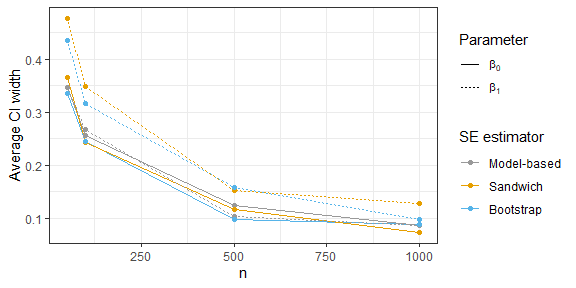plot_results("coverage", 3)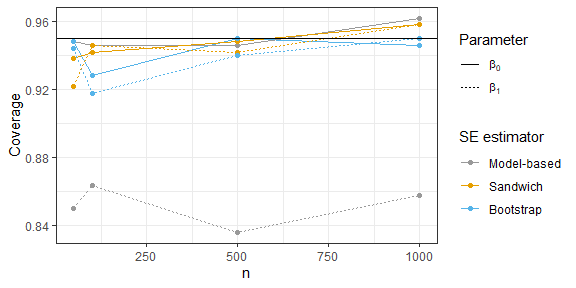Like the sandwich estimator, the bootstrap results in wider intervals for $$\beta_1$$, but is much closer to achieving 95% coverage compared to the model-based estimator.

# Statistical appendix

For notational simplicity, we build a matrix $$\mathbb{X}$$, whose first column is all 1’s (the intercept column) and whose second column is $$(X_1, \dots, X_n)^T$$. We also define a matrix

$\Sigma = \begin{pmatrix}\sigma^2_1 &\dots& 0 \\ \vdots &\ddots& \vdots \\ 0 & \dots & \sigma^2_n\end{pmatrix}$

The ordinary least squares estimator of $$\beta$$ is $$\hat{\beta} = (\mathbb{X}^T\mathbb{X})^{-1}\mathbb{X}^T\mathbb{Y}$$. The variance of this estimator, which we call $$\mathbb{V}$$ is

$\text{Var}(\hat{\beta}) = (\mathbb{X}^T\mathbb{X})^{-1}\mathbb{X}^T\Sigma\mathbb{X}(\mathbb{X}^T\mathbb{X})^{-1}$

The usual estimator of $$\mathbb{V}$$ is the model-based standard error $$s^2(\mathbb{X}^T\mathbb{X})^{-1}$$, where $$s^2 = \frac{\sum_i (Y_i - (\hat{\beta}_0 + \hat{\beta}_1X_i))^2}{n-1}$$. However, under our heteroskedastic model where the $$\sigma^2_i$$ are not all equal, this is not a consistent estimator of $$\mathbb{V}$$! That is to say, even in large samples, we cannot generally expect this estimator to be close to the truth.

A better estimator for our setting is the sandwich standard error, or Huber-White standard error, given by

$(\mathbb{X}^T\mathbb{X})^{-1}\mathbb{X}^T\hat{\Sigma}\mathbb{X}(\mathbb{X}^T\mathbb{X})^{-1}$

where

$\hat{\Sigma} = \begin{pmatrix}(Y_1 - (\hat{\beta}_0 + \hat{\beta}_1X_1))^2 &\dots& 0 \\ \vdots &\ddots& \vdots \\ 0 & \dots & (Y_n - (\hat{\beta}_0 + \hat{\beta}_1X_n))^2\end{pmatrix}$